# Velocity Acceleration and Motion A Velocity 1 How

• Slides: 16
Download presentationVelocity, Acceleration, and Motion A. Velocity 1. How fast an object is going in a given direction a. Ex – 30 miles per hour, east B. Acceleration 1. The change in either the speed of an object or its direction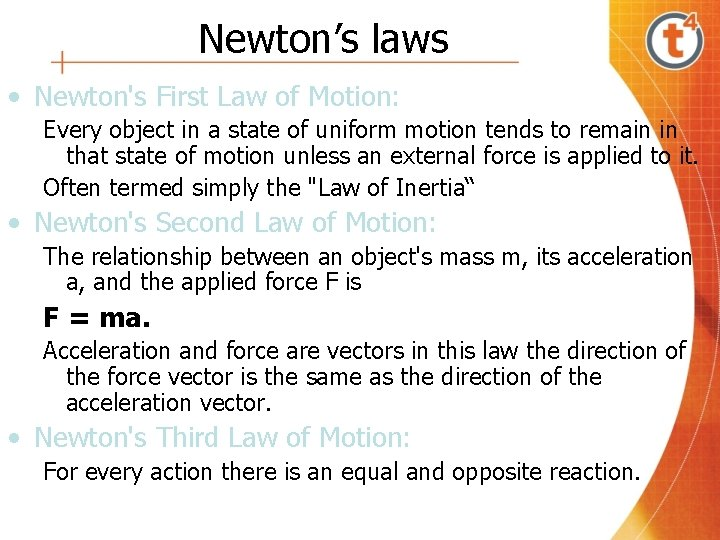Newton’s laws • Newton's First Law of Motion: Every object in a state of uniform motion tends to remain in that state of motion unless an external force is applied to it. Often termed simply the "Law of Inertia“ • Newton's Second Law of Motion: The relationship between an object's mass m, its acceleration a, and the applied force F is F = ma. Acceleration and force are vectors in this law the direction of the force vector is the same as the direction of the acceleration vector. • Newton's Third Law of Motion: For every action there is an equal and opposite reaction.Levers • Levers are one of the simple machines that were probably used in prehistoric times. Levers were first described about 260 BC by the ancient Greek mathematician Archimedes (287 -212 BC).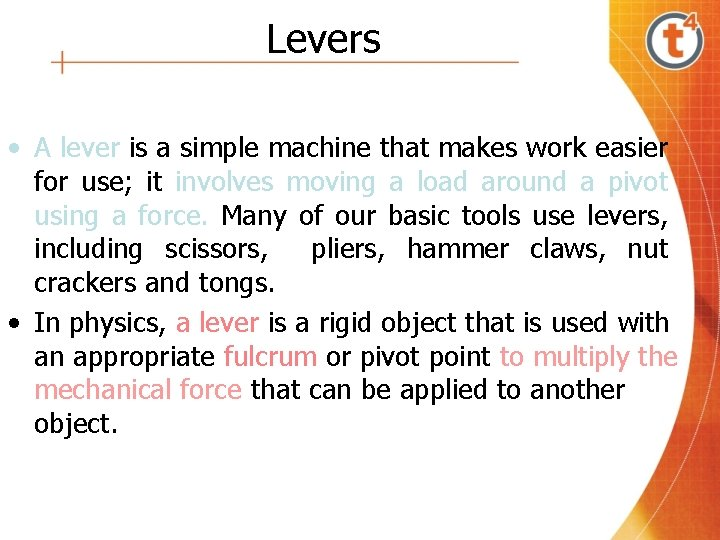Levers • A lever is a simple machine that makes work easier for use; it involves moving a load around a pivot using a force. Many of our basic tools use levers, including scissors, pliers, hammer claws, nut crackers and tongs. • In physics, a lever is a rigid object that is used with an appropriate fulcrum or pivot point to multiply the mechanical force that can be applied to another object.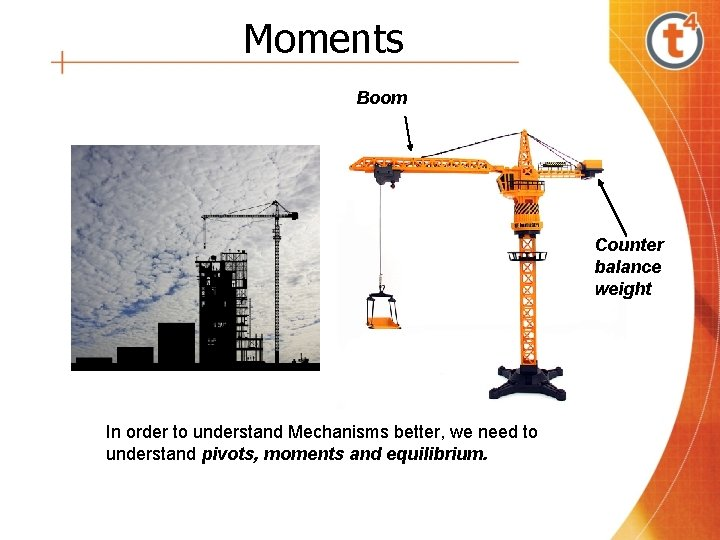Moments Boom Counter balance weight In order to understand Mechanisms better, we need to understand pivots, moments and equilibrium.Equilibrium When something is balanced it is said to be in equilibrium. Pivot point Or fulcrum FulcrumMoments The Moment of a Force is the force multiplied by the distance from the pivot point. Distance (d) Fulcrum Moment = F x d Force (F)Moments Torque may be represented as shown. Force Pivot Point Torque (turning force) = Force x Distance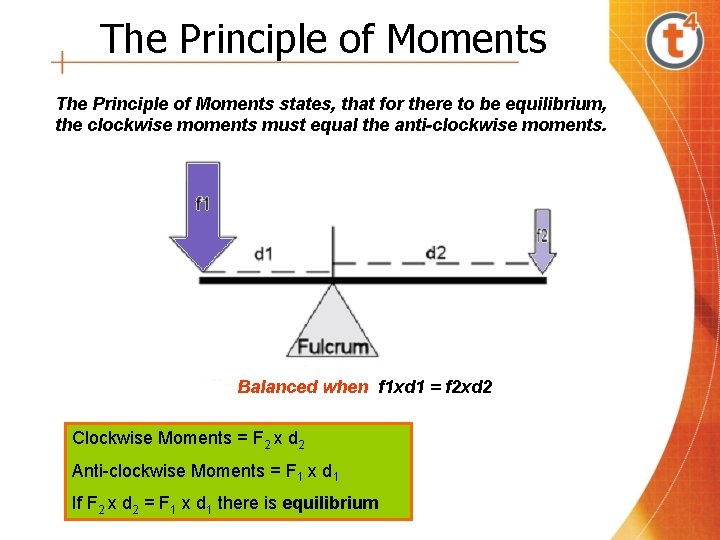The Principle of Moments states, that for there to be equilibrium, the clockwise moments must equal the anti-clockwise moments. Balanced when f 1 xd 1 = f 2 xd 2 Clockwise Moments = F 2 x d 2 Anti-clockwise Moments = F 1 x d 1 If F 2 x d 2 = F 1 x d 1 there is equilibrium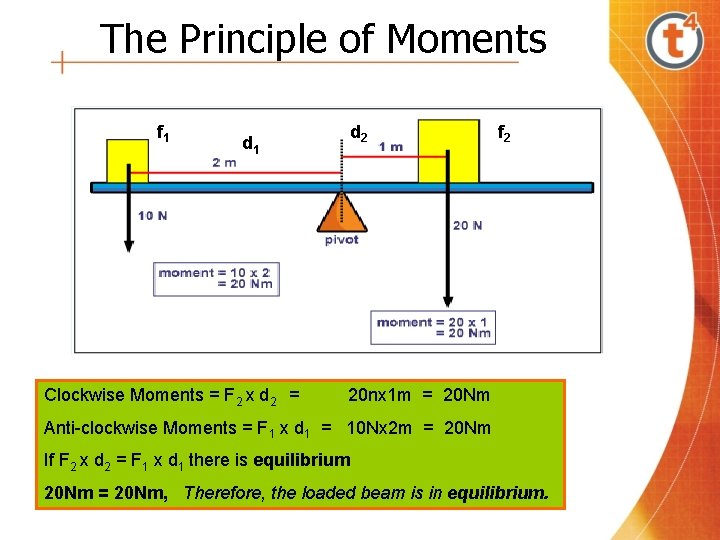The Principle of Moments f 1 d 1 Clockwise Moments = F 2 x d 2 = d 2 f 2 20 nx 1 m = 20 Nm Anti-clockwise Moments = F 1 x d 1 = 10 Nx 2 m = 20 Nm If F 2 x d 2 = F 1 x d 1 there is equilibrium 20 Nm = 20 Nm, Therefore, the loaded beam is in equilibrium.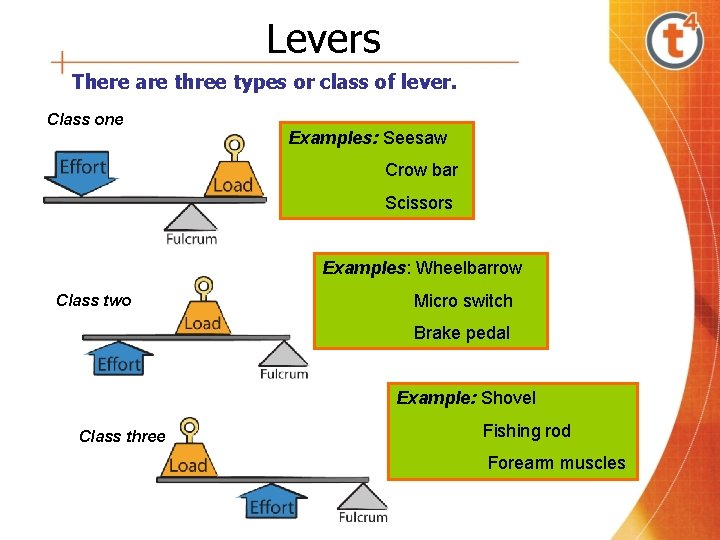Levers There are three types or class of lever. Class one Examples: Seesaw Crow bar Scissors Examples: Wheelbarrow Class two Micro switch Brake pedal Example: Shovel Class three Fishing rod Forearm musclesLevers Class One Levers See-saw Crowbar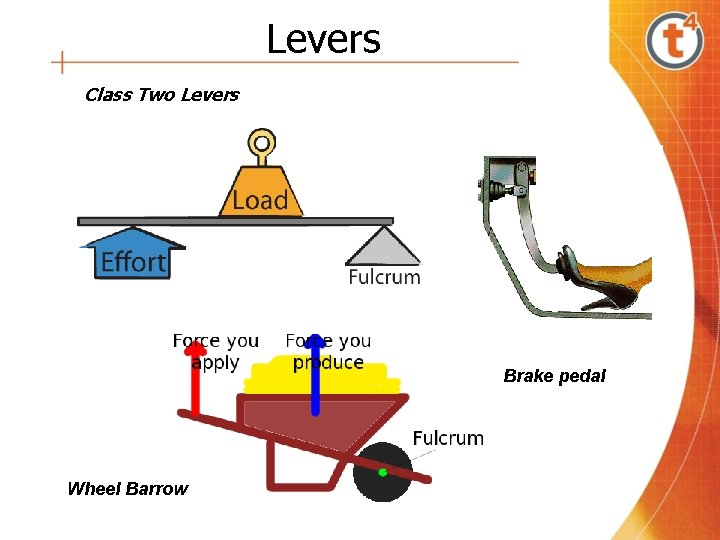Levers Class Two Levers Brake pedal Wheel Barrow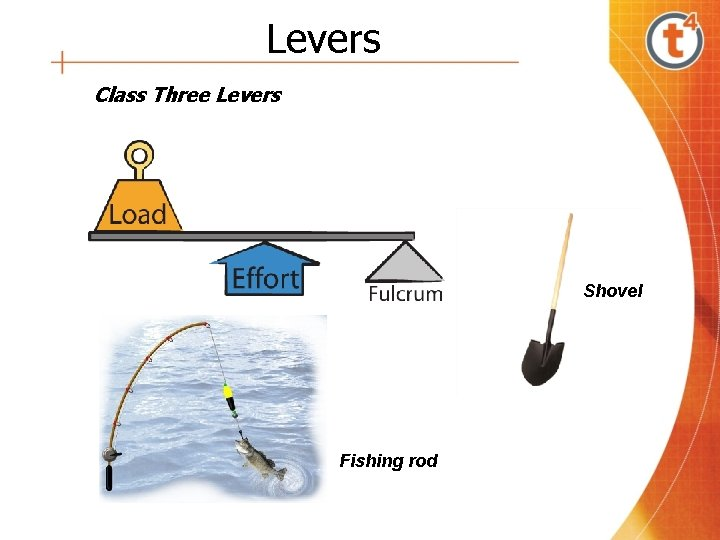Levers Class Three Levers Shovel Fishing rodMechanical Advantage = Load Effort Man lifting a Stone with a Lever Load Fulcrum Effort LeverMechanical Advantage - Calculation To raise a weight 400 N. Effort It was found that the weight could be lifted with an effort of 100 N. What is the Mechanical Advantage of the mechanism? Load Mechanical Advantage = Load Effort = 400 N = 4: 1 100 N or 4